``````library(swimplot)
library(ggplot2)
#> Warning: package 'ggplot2' was built under R version 4.0.3``````

# Introduction to swimmers plots

A swimmer plot is a graphical tool used to display individual trajectories over time.

A swimmer plot is able to tell a full story using horizontal bars to represent each subject (or study unit), while lines, points, and arrows are utilized to display additional information.

The “swimmer” package has a variety of functions which add layers to a swimmer plot by implementing ggplot functions.

This vignette goes through examples to create swimmers plots, and demonstrates converting a dataframe to the required format.

# A working example

## The Data and research question

• This is a clinical trial of 36 patients in which patients are randomized to one of two treatment arms, at 5 months patients are intended to switch arms, for each patient the adverse events and response information is recorded. The data is stored in three dataframes, `ClinicalTrial.Arm`, `ClinicalTrial.AE`, and `ClinicalTrial.Response`
``knitr::kable(head(ClinicalTrial.Arm,10))``
id Arm End_trt Continued_treatment Sex Age
1 Arm A 3.26 NA F >=65
2 Arm A 2.00 NA F <65
2 Off Treatment 10.00 NA F <65
2 Arm A 15.45 NA F <65
3 Arm B 5.00 NA F >=65
3 Arm A 14.84 NA F >=65
4 Arm B 3.51 NA F <65
5 Arm B 6.00 NA F >=65
5 Arm A 7.44 NA F >=65
6 Arm B 3.70 NA F <65
``knitr::kable(head(ClinicalTrial.AE,10))``
id time event Related Sex Age
33 2.20 AE Likely M >=65
14 1.00 SAE Possibly F <65
14 3.67 Death Not Likely F <65
3 14.58 AE Likely F >=65
29 5.44 SAE Possibly M >=65
21 0.50 AE Possibly M >=65
10 5.00 SAE Not Likely F <65
10 6.06 SAE Possibly F <65
2 2.00 SAE Not Likely F <65
3 14.84 Death Likely F >=65
``knitr::kable(head(ClinicalTrial.Response,10))``
id Response_start Response_end Response Continued_response Sex Age
10 3.74 6.02 CR NA F <65
11 1.48 2.21 PR NA F >=65
12 0.20 0.67 CR NA F <65
13 2.07 2.59 CR NA F >=65
15 0.35 4.05 PR NA M >=65
16 4.49 7.30 CR NA M <65
17 1.09 1.44 PR NA M >=65
18 0.55 1.32 CR NA M <65
19 0.00 0.96 PR 1 M >=65
2 3.36 8.77 PR NA F <65

## Basic plot

• The `swimmer_plot()` function creates the base of the swimmer plot
• The required arguments are a dataframe, an id column name, and the column name of where the bars end
• By default the bars are in increasing order, but any order can be specified
• A column name for the fill, transparency and colour (outline of the bars) can also be included
• Individual bars can change colour/transparency over time
• Other aesthetics can be manipulated using `geom_bar()` arguments (eg. fill,width, alpha)
``swimmer_plot(df=ClinicalTrial.Arm,id='id',end='End_trt',fill='lightblue',width=.85)``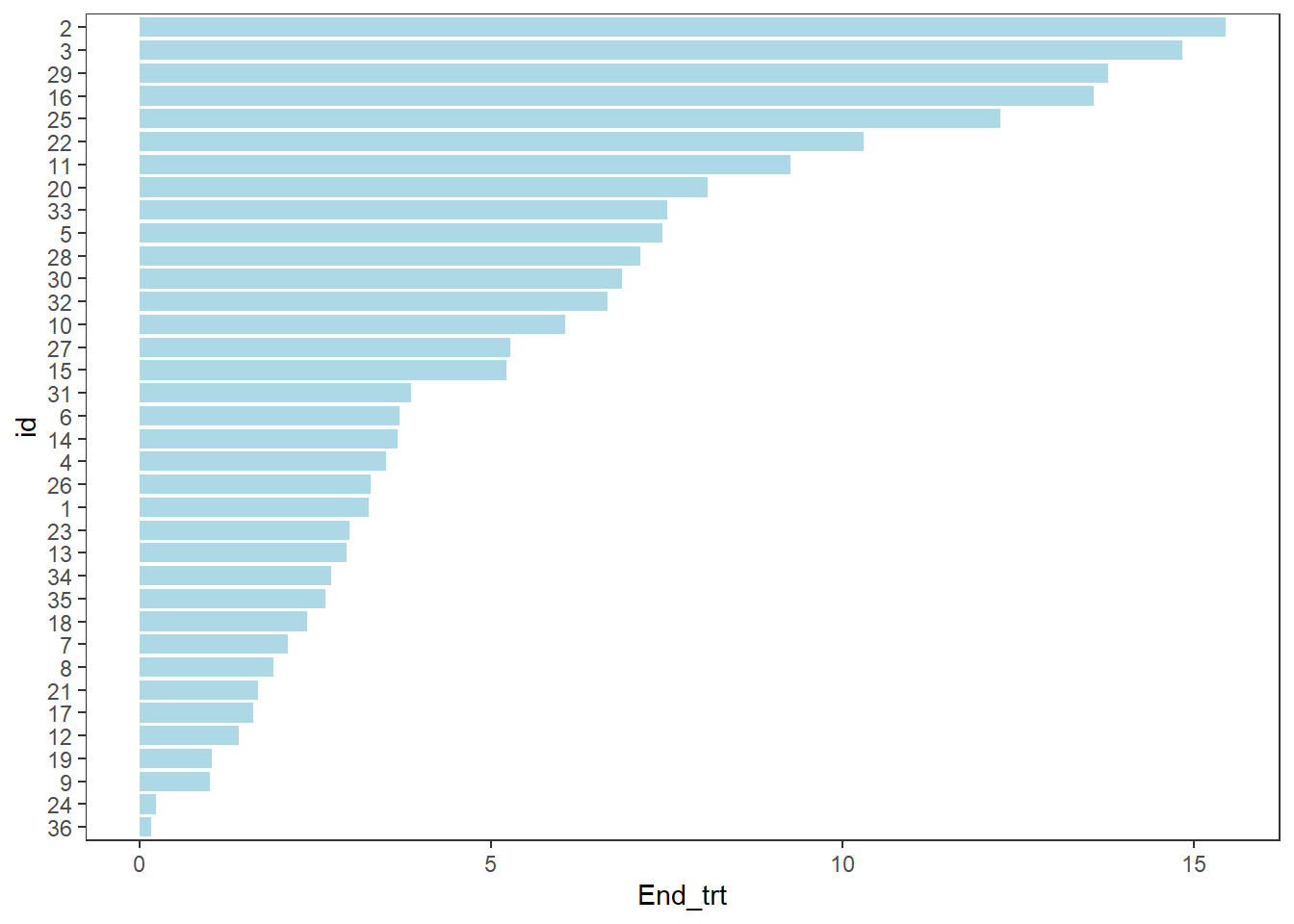## Modifying the order and colours of the bars

The `swimmer_plot()` function includes the option to have the bars change colours. Each section of the bars should be in a different row, where each row includes the time that section ends. By default the bars are plotted in increasing order, a column name can be used in the argument id_order to have the bars sorted first by a column, or string of IDs can be specified to have the bars in a specific order. Here the bars are ordered by the starting treatment, and follow up time.

``````arm_plot <- swimmer_plot(df=ClinicalTrial.Arm,id='id',end='End_trt',name_fill='Arm',
id_order='Arm',col="black",alpha=0.75,width=.8)

arm_plot``````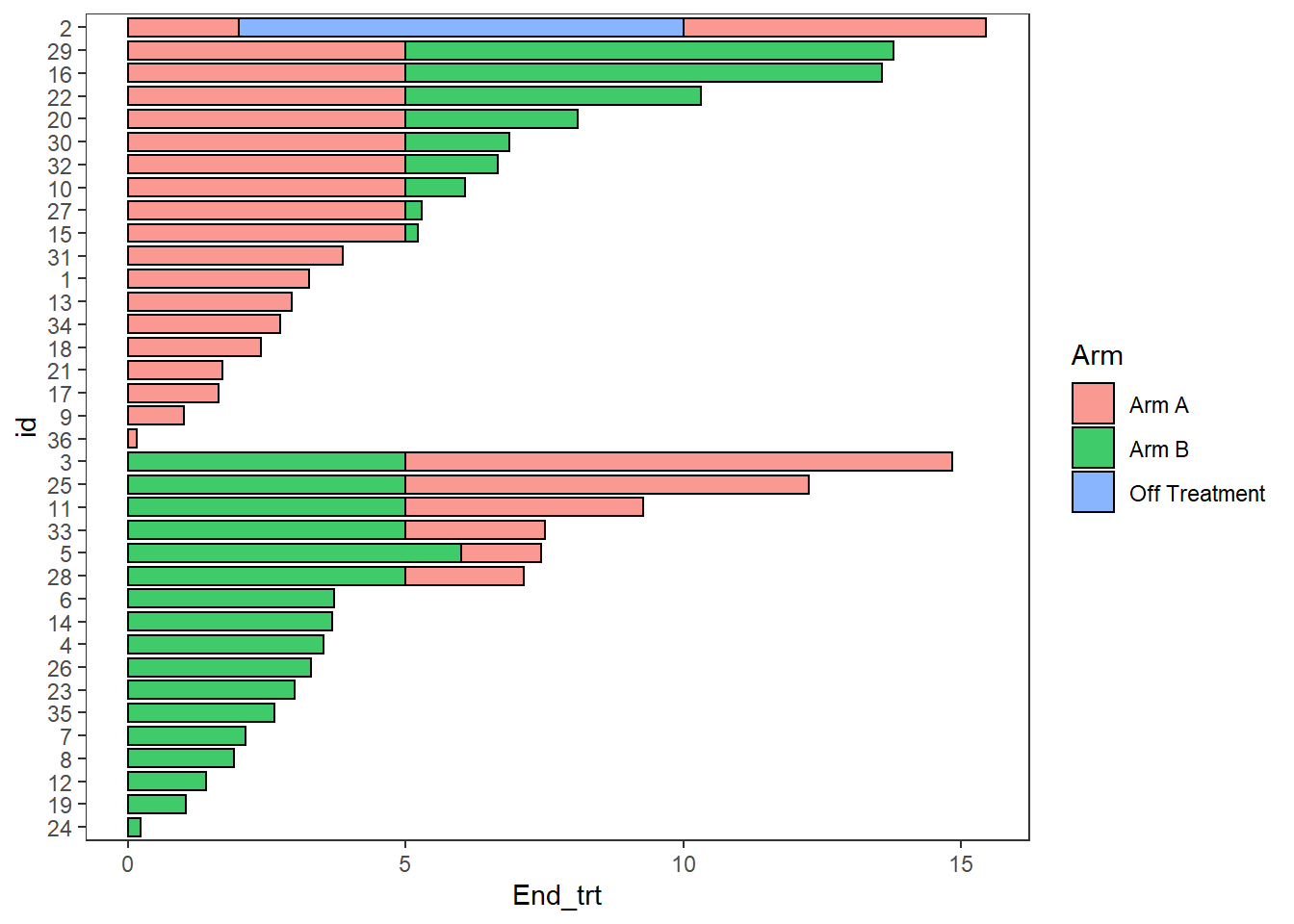## Stratification

Plots can be stratified by any variables in the dataframe

``````swim_plot_stratify <-swimmer_plot(df=ClinicalTrial.Arm,id='id',end='End_trt',name_fill='Arm',
col="black",alpha=0.75,width=.8,base_size = 14,stratify= c('Age','Sex'))

swim_plot_stratify``````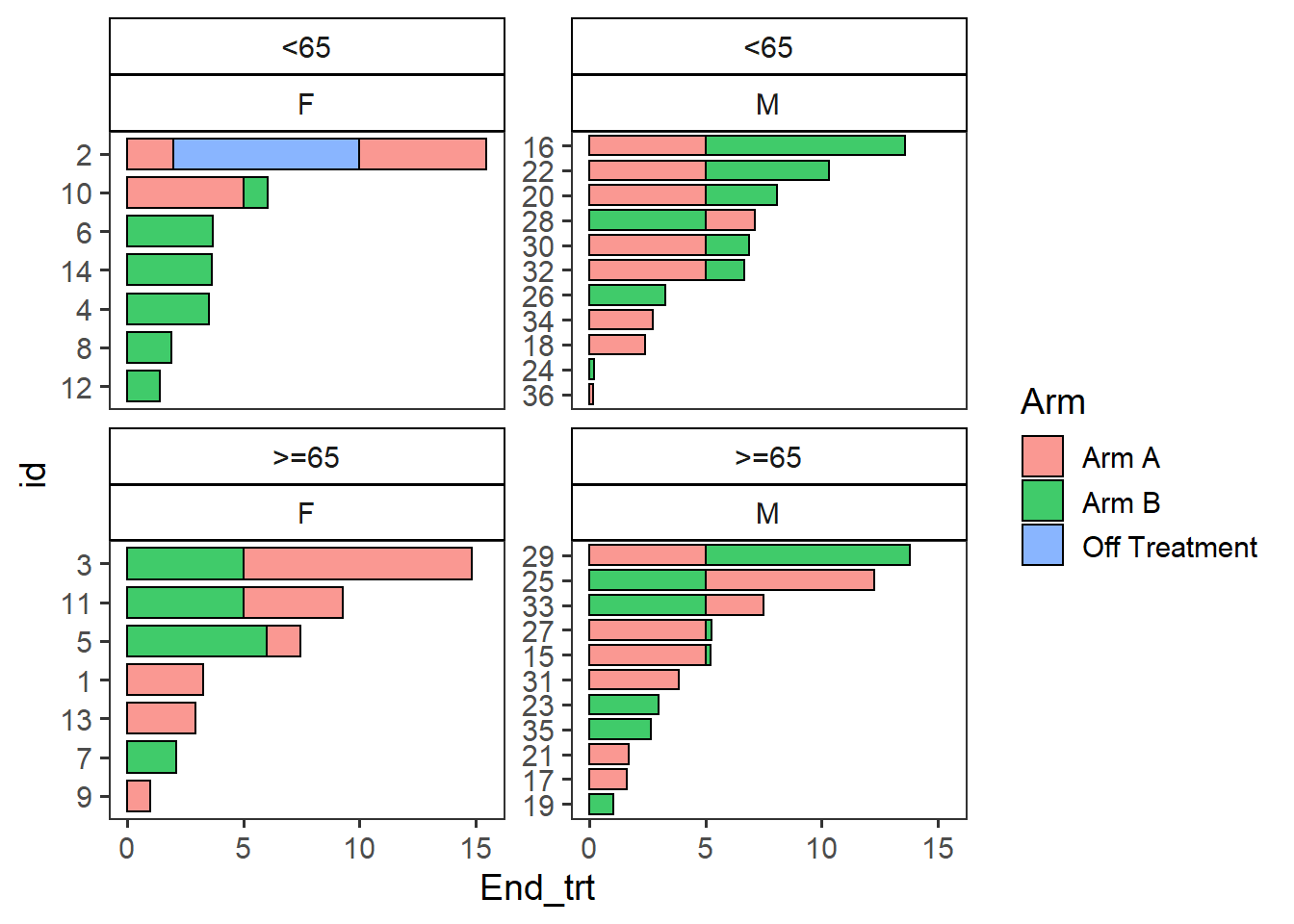• Points are added with the `swimmer_points()` function
• The required arguments are a dataframe, an id column name, and the column name of the point’s location
• The shape, size, fill, stroke, and transparency can all be mapped to columns
• The argument adj.y can be used to adjust the height position of points withing a bar
• Other aesthetics can be manipulated using `geom_point()` arguments
``````AE_plot <- arm_plot + swimmer_points(df_points=
ClinicalTrial.AE,id='id',time='time',name_shape =
'event',size=2.5,fill='white',col='black')
AE_plot``````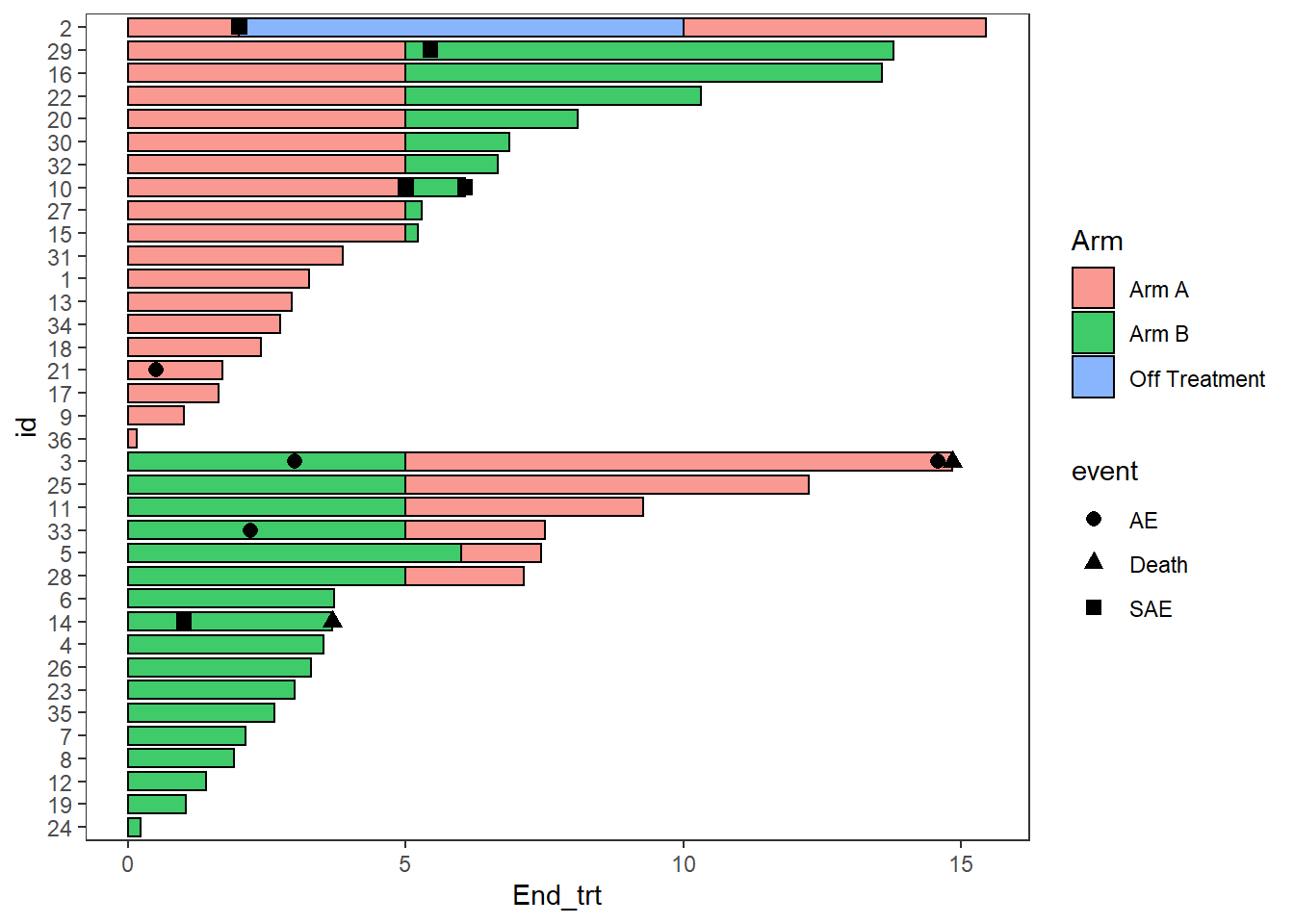Multiple aesthetics can be mapped to different columns

``````arm_plot + swimmer_points(df_points=
ClinicalTrial.AE,id='id',time='time',name_shape =
'event',size=2.5,fill='white',name_col = 'Related')``````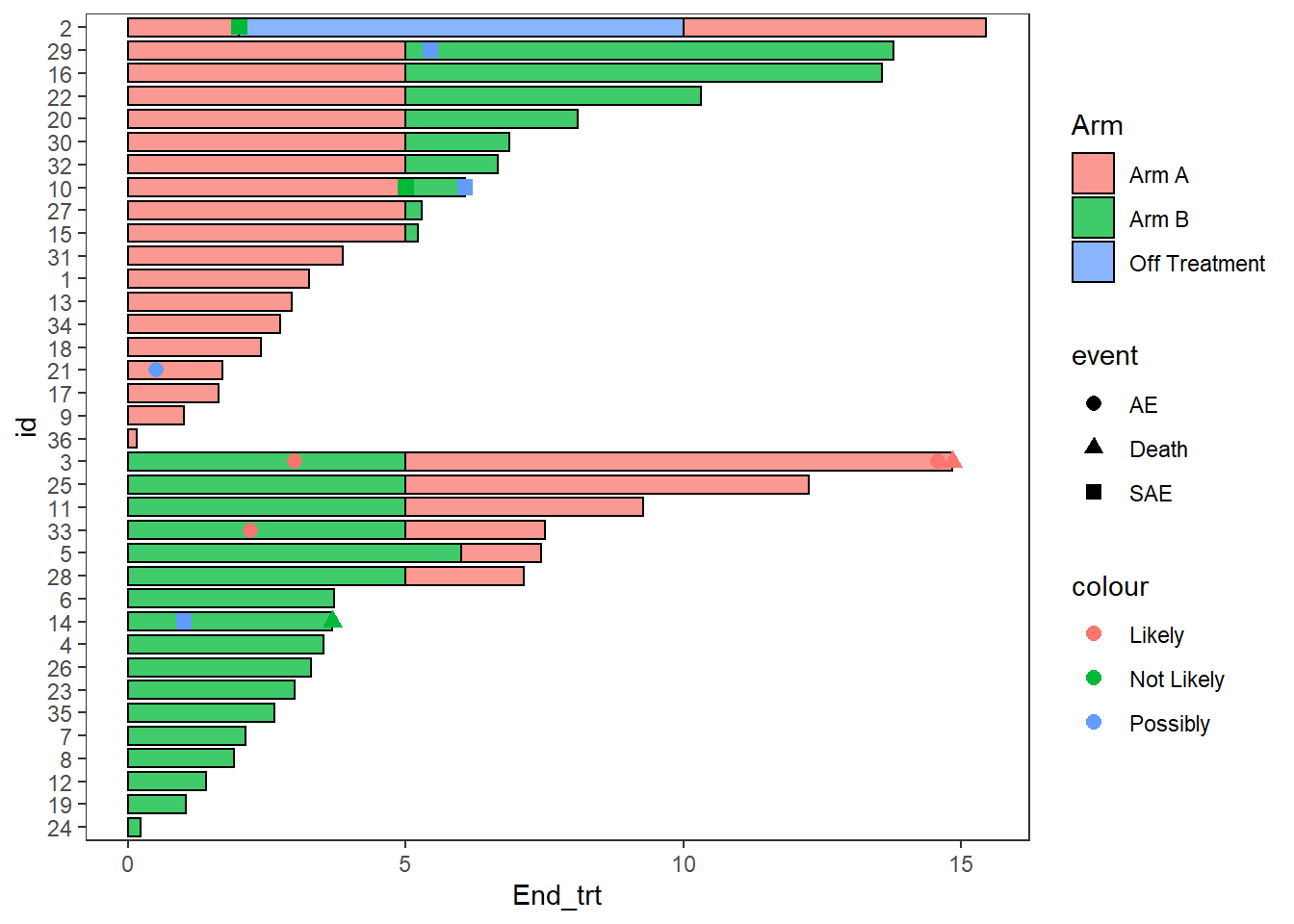• Lines are added with the `swimmer_lines()` function
• The required arguments are a dataframe, an id column name, and the column names of the line’s start and end locations
• The linetype, colour, size, and transparency can all be mapped to columns
• The argument adj.y can be used to adjust the height position of lines withing a bar
• Other aesthetics can be manipulated using `geom_segment()` arguments
``````Response_plot <- arm_plot +
swimmer_lines(df_lines=ClinicalTrial.Response,id='id',start =
'Response_start',end='Response_end',name_col='Response',size=1)

Response_plot``````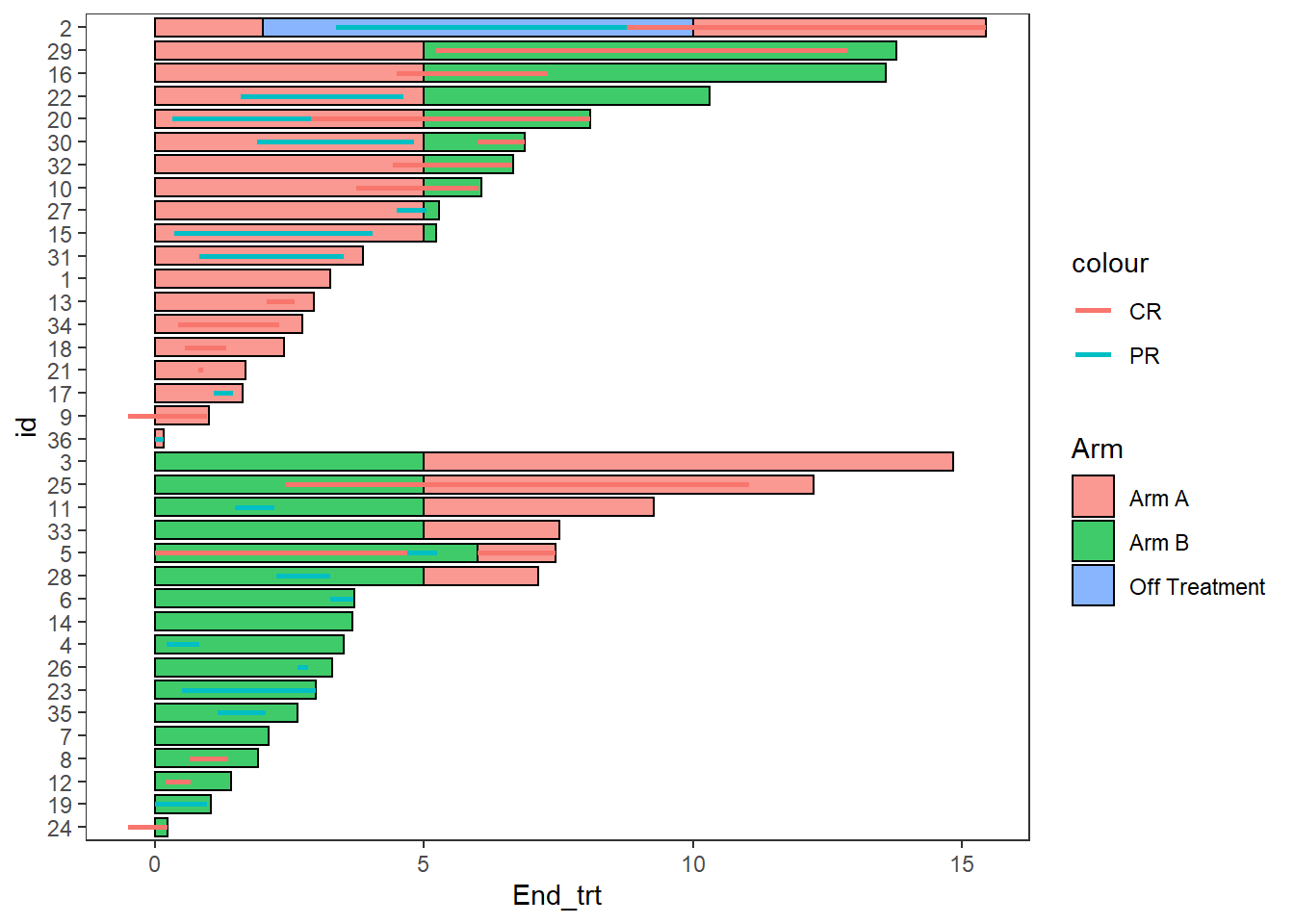## Adding lines and points together

• The function `swimmer_points_from_lines()` adds points to a plot at the start and end of each line
• The required arguments are the same as `swimmer_lines()`
• An additional argument “cont” can be used to specify lines which do not end
• Other aesthetics can be manipulated using `geom_point()` arguments
``````Response_plot_with_points <- Response_plot+
swimmer_points_from_lines(df_lines=ClinicalTrial.Response,id='id',start =
'Response_start',end = 'Response_end', cont =
'Continued_response',name_col='Response',size=2)

Response_plot_with_points``````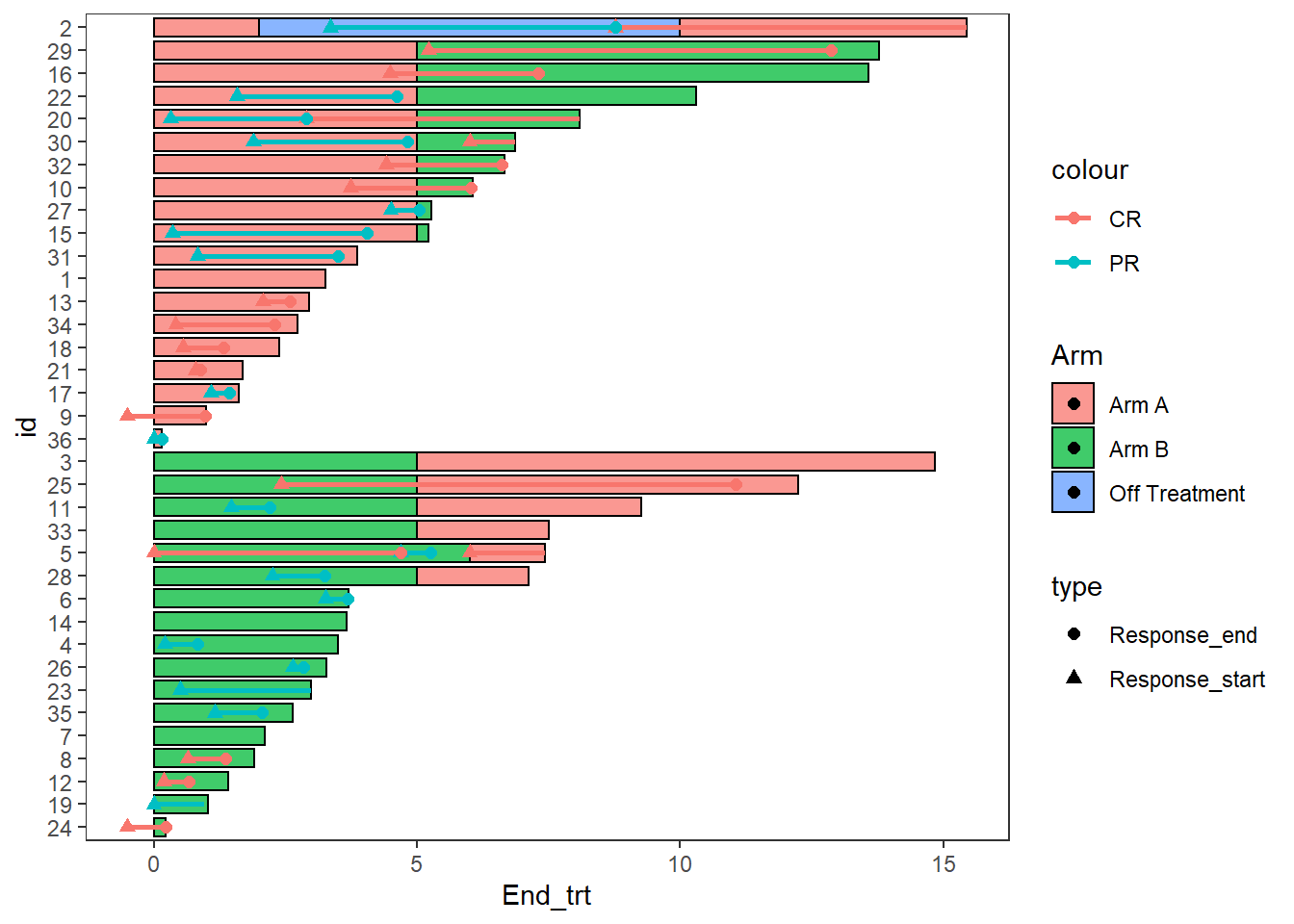• Arrows are added at the end of bars with the `swimmer_arrows()` function
• The required arguments are a dataframe, an id column name, and the column names of the location the arrows begin
• An additional argument “cont” can be used if only some bars will have an arrow
• The colour can be mapped to a column
• Features of the arrows which can be modified include the size, length and type of arrow
• Other aesthetics can be manipulated using `geom_segment()` arguments

The example below uses arrows to demonstrate patients remaining on treatment after the end of follow up

``````AE_plot+
swimmer_arrows(df_arrows=ClinicalTrial.Arm,id='id',arrow_start='End_trt',
cont = 'Continued_treatment',name_col='Arm',type =
"open",cex=1)``````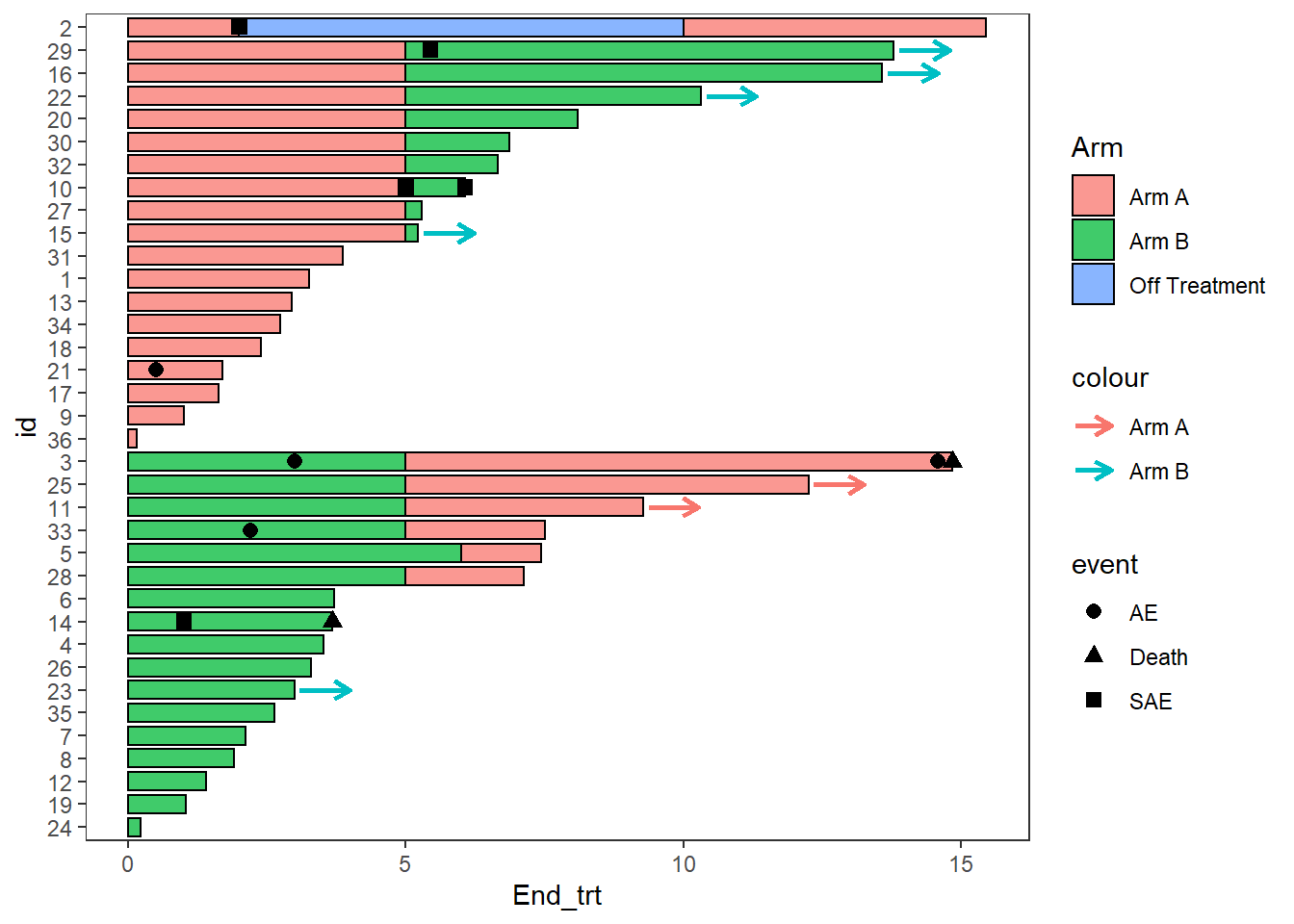Since none of the patients continue on “Off treatment” the arrow colours do not match the bars, this can be fixed by adding the layer `scale_color_discrete(drop=FALSE)`, the option show.legend = FALSE can also be employed as the arrow legend is not necessary

``````AE_plot <- AE_plot+
swimmer_arrows(df_arrows=ClinicalTrial.Arm,id='id',arrow_start='End_trt',
cont = 'Continued_treatment',name_col='Arm',show.legend = FALSE,type =
"open",cex=1) + scale_color_discrete(drop=FALSE)

AE_plot``````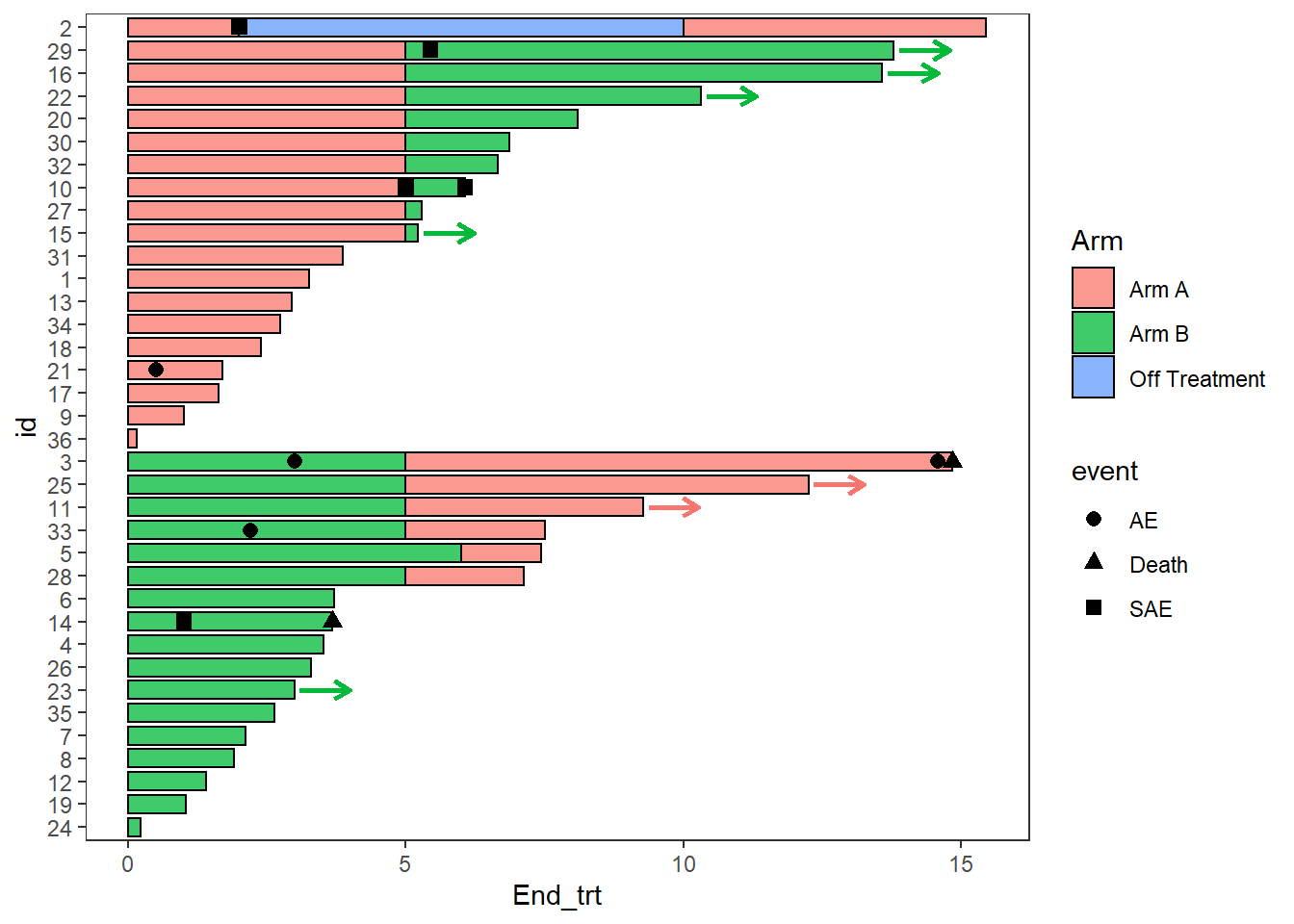Another arrow example, here the arrows are also used to demonstrate a continued treatment

``````Response_plot_with_points <- Response_plot_with_points+
swimmer_arrows(df_arrows=ClinicalTrial.Response,id='id',arrow_start='Response_end',
cont = 'Continued_response',name_col='Response',show.legend = FALSE,type =
"open",cex=1)

Response_plot_with_points``````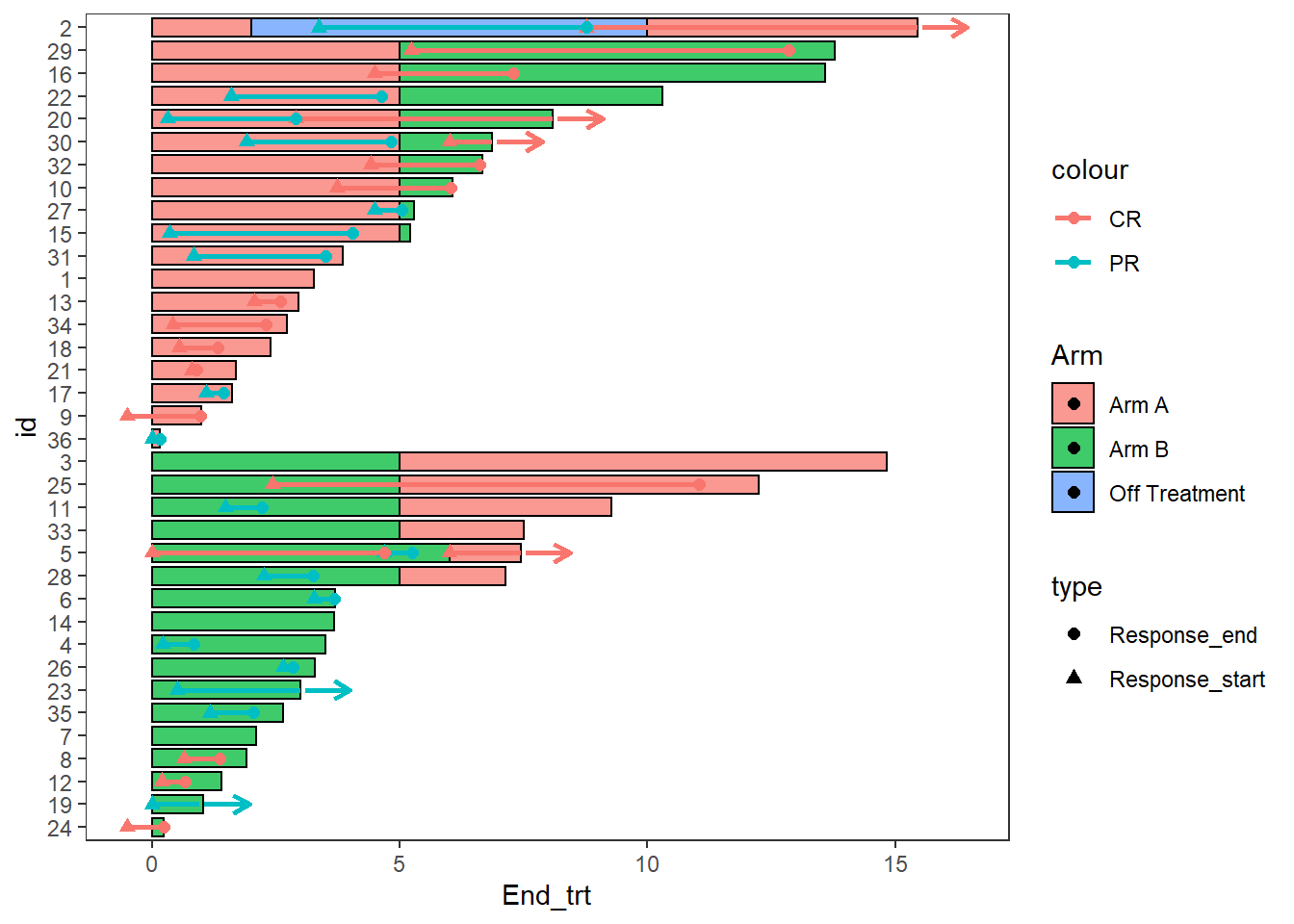## Making the plots more aesthetically pleasing with ggplot manipulations

### Modifying Colours and shapes

``````AE_plot <-  AE_plot +
scale_fill_manual(name="Treatment",values=c("Arm A" = "#e41a1c", "Arm B"="#377eb8","Off Treatment"='#4daf4a'))+
scale_color_manual(name="Treatment",values=c("Arm A"="#e41a1c", "Arm B" ="#377eb8","Off Treatment"='#4daf4a')) +

AE_plot``````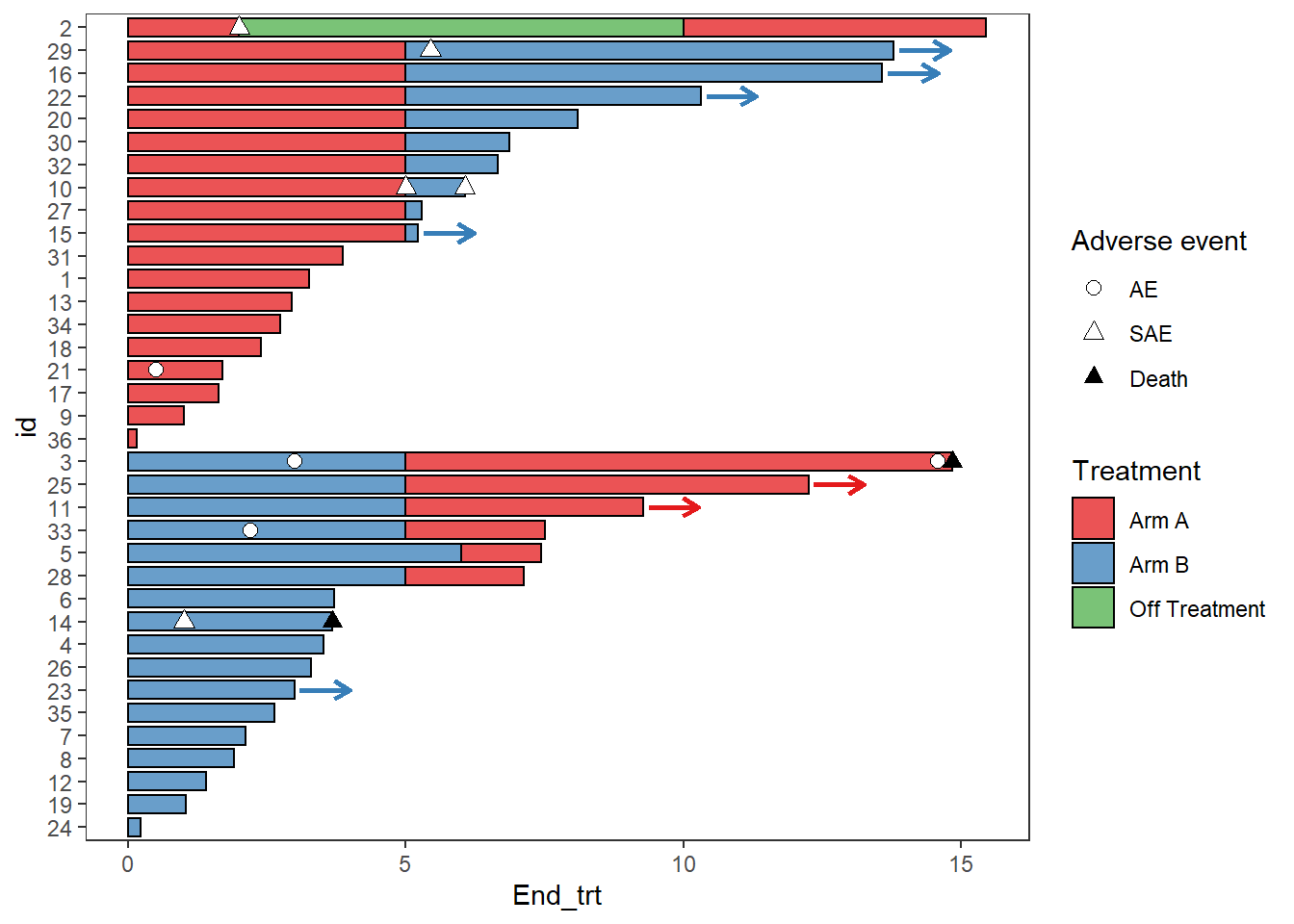``````Response_plot_with_points <- Response_plot_with_points +
scale_fill_manual(name="Treatment",values=c("Arm A" ="#e41a1c", "Arm B"="#377eb8","Off Treatment"='#4daf4a'))+
scale_color_manual(name="Response",values=c("grey20","grey80"))+
scale_shape_manual(name='',values=c(17,15),breaks=c('Response_start','Response_end'),
labels=c('Response start','Response end'))

Response_plot_with_points``````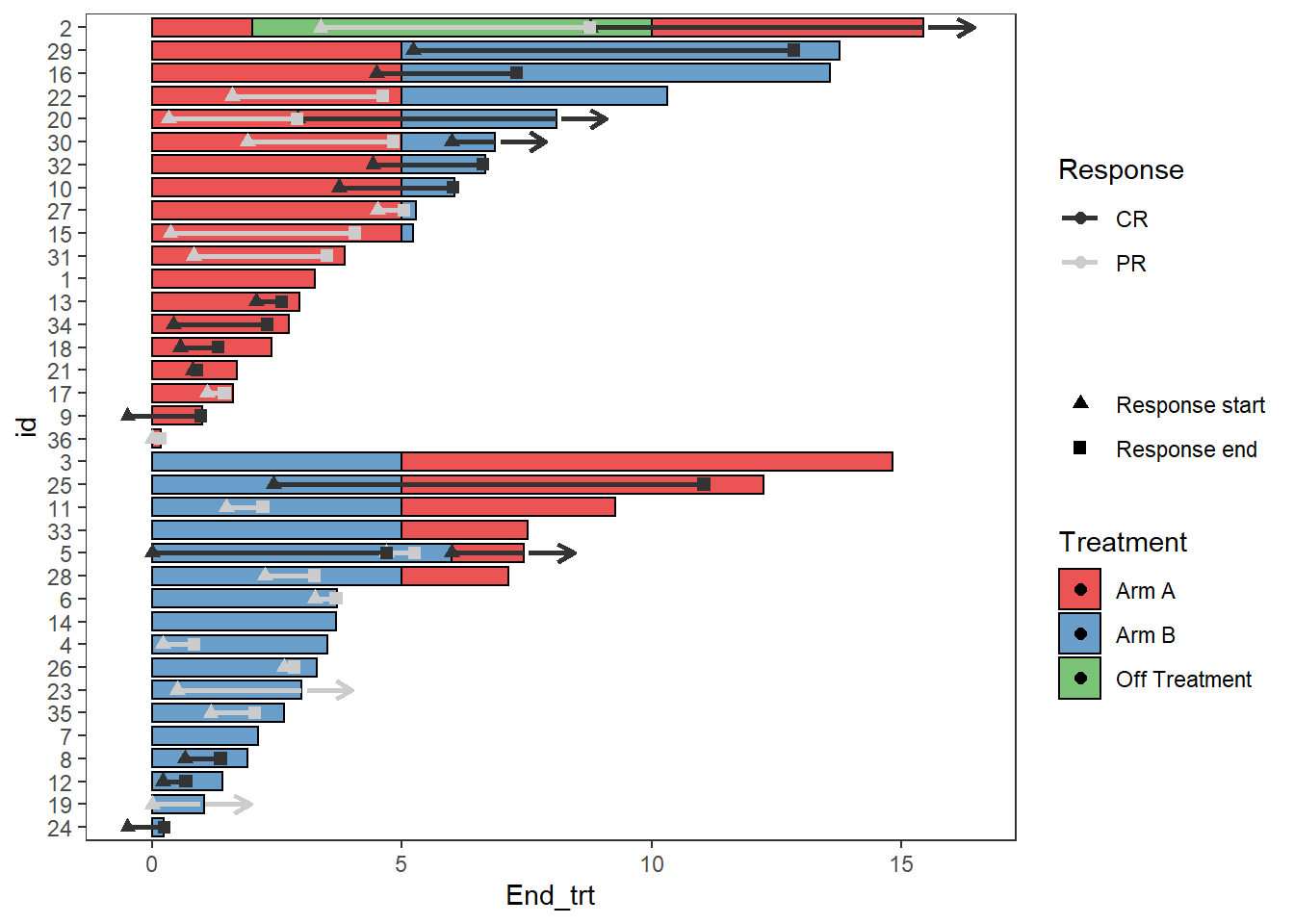### Legends

Sometimes there will be points within the fill of the legend, this can be turned off with the layer `guides()`

``````
Response_plot_with_points <- Response_plot_with_points+guides(fill = guide_legend(override.aes = list(shape = NA)))
Response_plot_with_points``````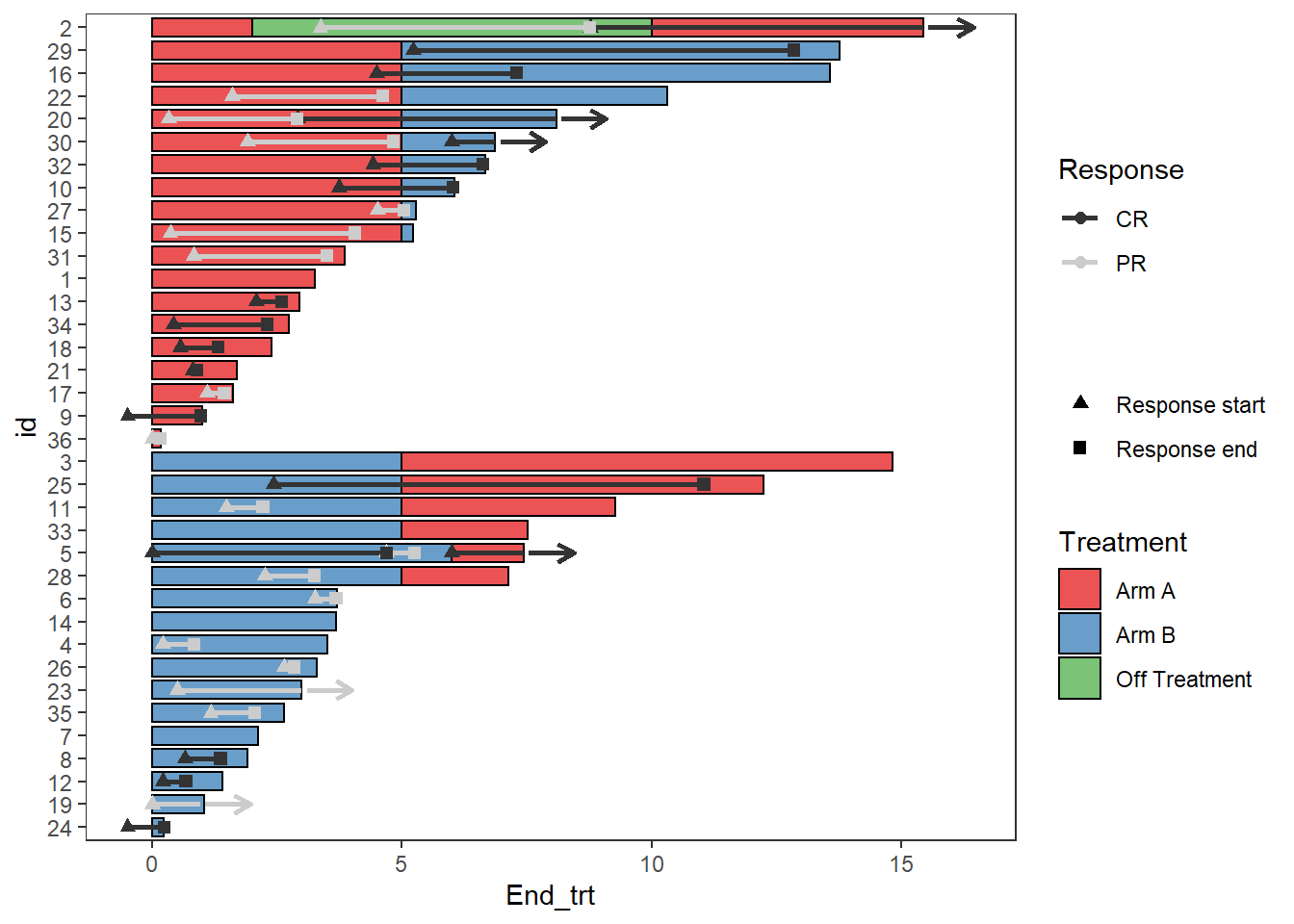### Add arrows to the legend

A work around to add arrows to the legend is using the symbol for an arrow within `annotate()`

``````
Response_plot_with_points <- Response_plot_with_points+
annotate("text", x=3.5, y=20.45, label="Continued response",size=3.25)+
annotate("text",x=2.5, y=20.25, label=sprintf('\u2192'),size=8.25)+
coord_flip(clip = 'off', ylim = c(0, 17))
Response_plot_with_points``````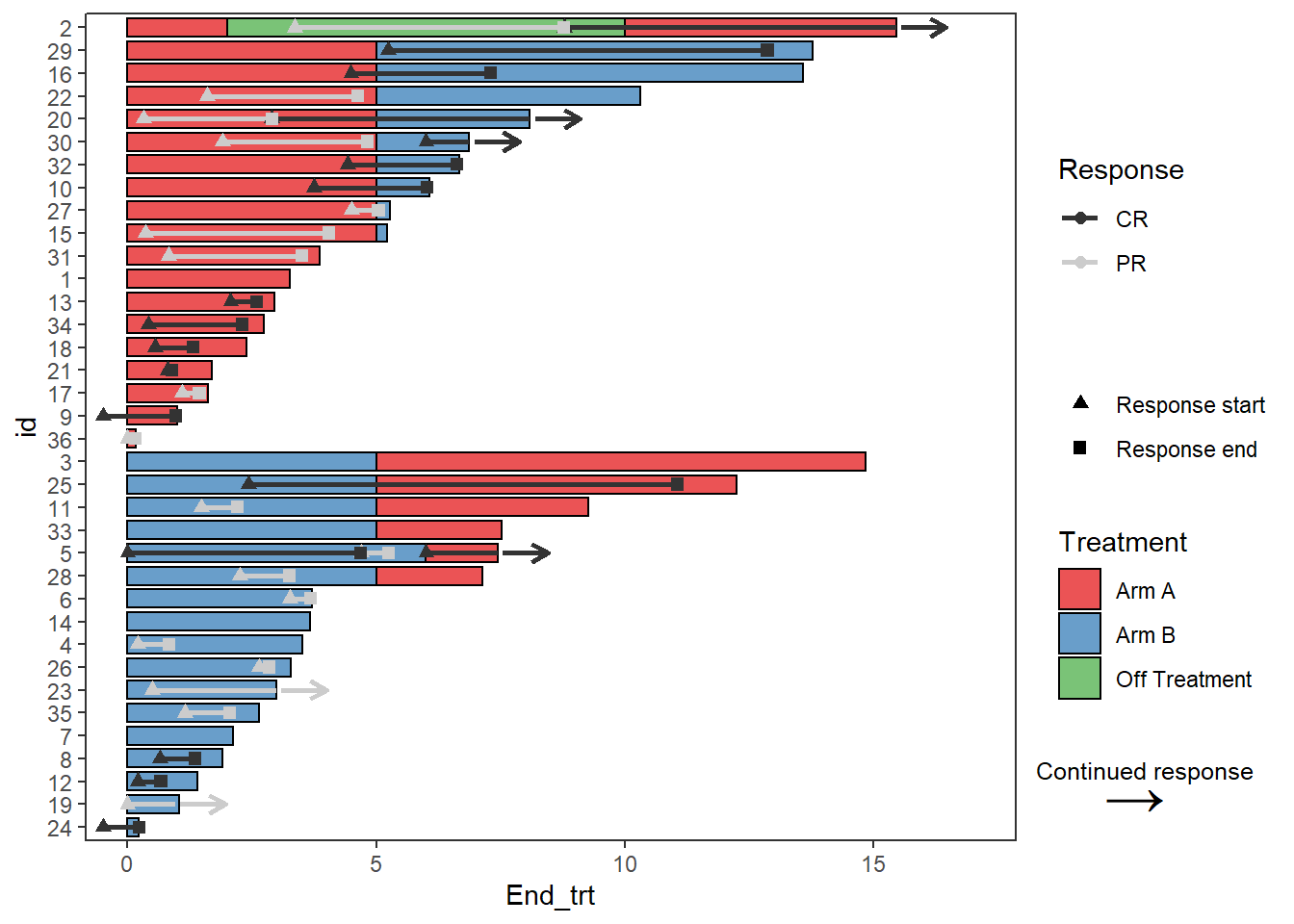### axis

The swimmer plot is a bar plot that has been turned on its side, so to modify the x axis it is actually required to change the y axis. This is also the case for adding axis labels

``````
Response_plot_with_points +  scale_y_continuous(name = "Time since enrollment (months)",breaks = seq(0,18,by=3))``````